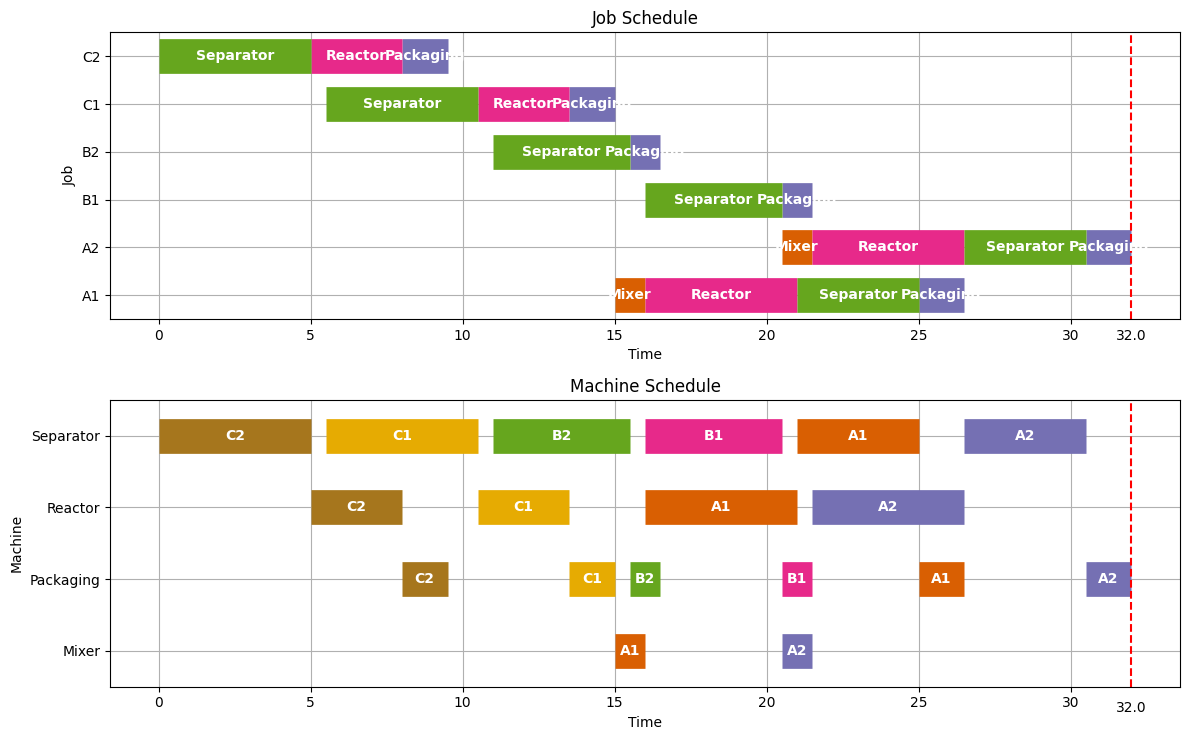# Extra material: Job shop scheduling#

# install dependencies and select solver
%pip install -q amplpy matplotlib

SOLVER = "highs"

from amplpy import AMPL, ampl_notebook

ampl = ampl_notebook(
modules=["highs"],  # modules to install
)  # instantiate AMPL object and register magics


## Job Shop Scheduling#

Job shop scheduling is one of the classic problems in Operations Research.

A job shop refers to a collection of machines that process jobs. The jobs may be simple or complex, such as the production of a custom part, a print job at printing house, a patient treated in a hospital, or a meal produced in a fast food restaurant. The term “machines” can refer any device, person, or resource involved in the processing the jobs. In what follows in this notebook, a job comprises a series of tasks that requiring use of particular machines for known duration, and which must be completed in specified order.

The job shop scheduling problem is to schedule a set of jobs on the available machines to optimize a metric of productivity. A typical metric is the makespan which refers to the time needed to process all jobs.

At a basic level, the data required to specify a job shop scheduling problem consists of two tables. The first table is a decomposition of the jobs into a series of tasks, each task listing the job name, name of the required machine, and task duration. A second table list task pairs where the first task must be completed before the second task can be started.

Real applications of job shop scheduling often include additional considerations such as time that may be required to set up machines prior to the start of the next job, or limits on the time a job can wait between processing steps.

## Job shop example#

The following example of a job shop is from from Christelle Gueret, Christian Prins, Marc Sevaux, “Applications of Optimization with Xpress-MP,” Dash Optimization, 2000.

In this example, there are three printed paper products that must pass through color printing presses in a particular order. The given data consists of a flow sheet showing the order in which each job passes through the color pressesand a table of data showing, in minutes, the amount of time each job requires on each machine.

Machine

Color

Paper 1

Paper 2

Paper 3

1

Blue

45

20

12

2

Green

-

10

17

3

Yellow

10

34

28

What is the minimum amount of time (i.e, what is the makespan) for this set of jobs?

The first step in the analysis is to decompose the process into a series of tasks. Each task c(job, machine) pair. Some tasks cannot start until a prerequisite task is completed.

Duration

(Paper 1, Blue)

45

-

(Paper 1, Yellow)

10

(Paper 1,Blue)

(Paper 2, Blue)

20

(Paper 2, Green)

(Paper 2, Green)

10

-

(Paper 2, Yellow)

34

(Paper 2, Blue)

(Paper 3, Blue)

12

(Paper 3, Yellow)

(Paper 3, Green)

17

(Paper 3, Blue)

(Paper 3, Yellow)

28

-

We convert this to a JSON style representation where tasks are denoted by (Job,Machine) tuples in Python. The task data is stored in a Python dictionary indexed by (Job,Machine) tuples. The task data consists of a dictionary with duration (‘dur’) and (Job,Machine) pair for any prerequisite task.

TASKS = {
("Paper_1", "Blue"): {"dur": 45, "prec": None},
("Paper_1", "Yellow"): {"dur": 10, "prec": ("Paper_1", "Blue")},
("Paper_2", "Blue"): {"dur": 20, "prec": ("Paper_2", "Green")},
("Paper_2", "Green"): {"dur": 10, "prec": None},
("Paper_2", "Yellow"): {"dur": 34, "prec": ("Paper_2", "Blue")},
("Paper_3", "Blue"): {"dur": 12, "prec": ("Paper_3", "Yellow")},
("Paper_3", "Green"): {"dur": 17, "prec": ("Paper_3", "Blue")},
("Paper_3", "Yellow"): {"dur": 28, "prec": None},
}


## Model formulation#

Each task is indexed by an ordered pair $$(j, m)$$ where $$j$$ refers to a job, and $$m$$ refers to a machine. Associated with each task is data describing the time needed to perform the task, and a preceding task that must be completed before the index task can start.

Parameter

Description

$$\text{dur}_{j,m}$$

Duration of task $$(j,m)$$

$$\text{prec}_{j,m}$$

A task $$(k,n) = \text{prec}_{j,m}$$ that must be completed before task $$(j,m)$$

The choice of decision variables for this problem are key to modeling. We introduce $$makespan$$ as the time needed to complete all tasks. $$makespan$$ is a candidate objective function. Variable $$start_{j,m}$$ denotes the time when task $$(j,m)$$ begins.

Decision Variables

Description

$$\text{makespan}$$

Completion of all jobs

$$\text{start}_{j,m}$$

Start time for task $$(j,m)$$

The constraints include lower bounds on the start and an upper bound on the completion of each task $$(j,m)$$

\begin{split} \begin{align} \text{start}_{j,m} & \geq 0\\ \text{start}_{j,m}+\text{dur}_{j,m} & \leq \text{makespan} \end{align} \end{split}

Any preceding tasks must be completed before task $$(j,m)$$ can start.

\begin{align} \text{start}_{k,n}+\text{dur}_{k,n}\leq\text{start}_{j,m}\ \ \ \ \text{for } (k,n) =\text{prec}_{j,m} \end{align}

Finally, for every task performed on machine $$m$$, there can be no overlap among those tasks. This leads to a set of pair-wise disjunctive constraints for each machine.

\begin{align} \left[\text{start}_{j,m}+\text{dur}_{j,m} \leq \text{start}_{k,m}\right] \veebar \left[\text{start}_{k,m}+\text{dur}_{k,m} \leq \text{start}_{j,m}\right] \end{align}

avoids conflicts for use of the same machine.

## AMPL implementation#

The job shop scheduling problem is implemented below in AMPL. The implementation consists of a function jobshop_model(TASKS) that accepts a dictionary of tasks and returns an AMPL model.

%%writefile jobshop_model.mod

set JOBS;
set MACHINES;
set DISJUNCTIONS within {JOBS, JOBS, MACHINES};

param ub;

var makespan >= 0, <= ub;
var start{TASKS} >= 0, <= ub;

minimize minimize_makespan: makespan;

start[j, m] + dur[j, m] <= makespan;

s.t. preceding {(j, m, k, n) in TASKORDER}:
start[k, n] + dur[k, n] <= start[j, m];

s.t. no_overlap {(j, k, m) in DISJUNCTIONS}:
(start[j, m] + dur[j, m] <= start[k, m]
or
start[k, m] + dur[k, m] <= start[j, m])
and not
(start[j, m] + dur[j, m] <= start[k, m]
and
start[k, m] + dur[k, m] <= start[j, m]);

Overwriting jobshop_model.mod

def jobshop_model(TASKS):
m = AMPL()

jobs = list(set([j for (j, m) in TASKS]))
machines = list(set([m for (j, m) in TASKS]))
(j, m, k, n)
if (k, n) == TASKS[(j, m)]["prec"]
]
disjunctions = [
(j, k, m) for (j, m) in tasks for (k, n) in tasks if m == n and j < k
]
ub = sum(dur[i] for i in dur)

m.set["JOBS"] = jobs
m.set["MACHINES"] = machines
m.set["DISJUNCTIONS"] = disjunctions

m.param["dur"] = dur
m.param["ub"] = ub

return m


<amplpy.ampl.AMPL at 0x7f54c3021600>

def jobshop_solve(model):
model.option["solver"] = SOLVER
model.solve()

start = model.var["start"].to_dict()
dur = model.param["dur"].to_dict()

results = [
{
"Job": j,
"Machine": m,
"Start": start[j, m],
"Duration": dur[j, m],
"Finish": start[j, m] + dur[j, m],
}
]
return results

results

HiGHS 1.5.1: HiGHS 1.5.1: optimal solution; objective 97
32 simplex iterations
0 branching nodes

[{'Job': 'Paper_1',
'Machine': 'Blue',
'Start': 41.99999999999993,
'Duration': 45.0,
'Finish': 86.99999999999993},
{'Job': 'Paper_1',
'Machine': 'Yellow',
'Start': 86.99999999999993,
'Duration': 10.0,
'Finish': 96.99999999999993},
{'Job': 'Paper_2',
'Machine': 'Blue',
'Start': 9.999999999999972,
'Duration': 20.0,
'Finish': 29.99999999999997},
{'Job': 'Paper_2',
'Machine': 'Green',
'Start': 0.0,
'Duration': 10.0,
'Finish': 10.0},
{'Job': 'Paper_2',
'Machine': 'Yellow',
'Start': 29.99999999999997,
'Duration': 34.0,
'Finish': 63.99999999999997},
{'Job': 'Paper_3',
'Machine': 'Blue',
'Start': 29.99999999999997,
'Duration': 12.0,
'Finish': 41.99999999999997},
{'Job': 'Paper_3',
'Machine': 'Green',
'Start': 79.99999999999997,
'Duration': 17.0,
'Finish': 96.99999999999997},
{'Job': 'Paper_3',
'Machine': 'Yellow',
'Start': 1.999999999999972,
'Duration': 28.0,
'Finish': 29.99999999999997}]


## Printing schedules#

import pandas as pd

schedule = pd.DataFrame(results)

print("\nSchedule by Job")
print(schedule.sort_values(by=["Job", "Start"]).set_index(["Job", "Machine"]))

print("\nSchedule by Machine")
print(schedule.sort_values(by=["Machine", "Start"]).set_index(["Machine", "Job"]))

Schedule by Job
Start  Duration  Finish
Job     Machine
Paper_1 Blue      42.0      45.0    87.0
Yellow    87.0      10.0    97.0
Paper_2 Green      0.0      10.0    10.0
Blue      10.0      20.0    30.0
Yellow    30.0      34.0    64.0
Paper_3 Yellow     2.0      28.0    30.0
Blue      30.0      12.0    42.0
Green     80.0      17.0    97.0

Schedule by Machine
Start  Duration  Finish
Machine Job
Blue    Paper_2   10.0      20.0    30.0
Paper_3   30.0      12.0    42.0
Paper_1   42.0      45.0    87.0
Green   Paper_2    0.0      10.0    10.0
Paper_3   80.0      17.0    97.0
Yellow  Paper_3    2.0      28.0    30.0
Paper_2   30.0      34.0    64.0
Paper_1   87.0      10.0    97.0


## Visualizing Results with Gantt Charts#

import matplotlib.pyplot as plt
import matplotlib as mpl

def visualize(results):
schedule = pd.DataFrame(results)
JOBS = sorted(list(schedule["Job"].unique()))
MACHINES = sorted(list(schedule["Machine"].unique()))
makespan = schedule["Finish"].max()

bar_style = {"alpha": 1.0, "lw": 25, "solid_capstyle": "butt"}
text_style = {"color": "white", "weight": "bold", "ha": "center", "va": "center"}
colors = mpl.cm.Dark2.colors

schedule.sort_values(by=["Job", "Start"])
schedule.set_index(["Job", "Machine"], inplace=True)

fig, ax = plt.subplots(2, 1, figsize=(12, 5 + (len(JOBS) + len(MACHINES)) / 4))

for jdx, j in enumerate(JOBS, 1):
for mdx, m in enumerate(MACHINES, 1):
if (j, m) in schedule.index:
xs = schedule.loc[(j, m), "Start"]
xf = schedule.loc[(j, m), "Finish"]
ax.plot([xs, xf], [jdx] * 2, c=colors[mdx % 7], **bar_style)
ax.text((xs + xf) / 2, jdx, m, **text_style)
ax.plot([xs, xf], [mdx] * 2, c=colors[jdx % 7], **bar_style)
ax.text((xs + xf) / 2, mdx, j, **text_style)

ax.set_title("Job Schedule")
ax.set_ylabel("Job")
ax.set_title("Machine Schedule")
ax.set_ylabel("Machine")

for idx, s in enumerate([JOBS, MACHINES]):
ax[idx].set_ylim(0.5, len(s) + 0.5)
ax[idx].set_yticks(range(1, 1 + len(s)))
ax[idx].set_yticklabels(s)
ax[idx].text(
makespan,
ax[idx].get_ylim() - 0.2,
"{0:0.1f}".format(makespan),
ha="center",
va="top",
)
ax[idx].plot([makespan] * 2, ax[idx].get_ylim(), "r--")
ax[idx].set_xlabel("Time")
ax[idx].grid(True)

fig.tight_layout()

visualize(results)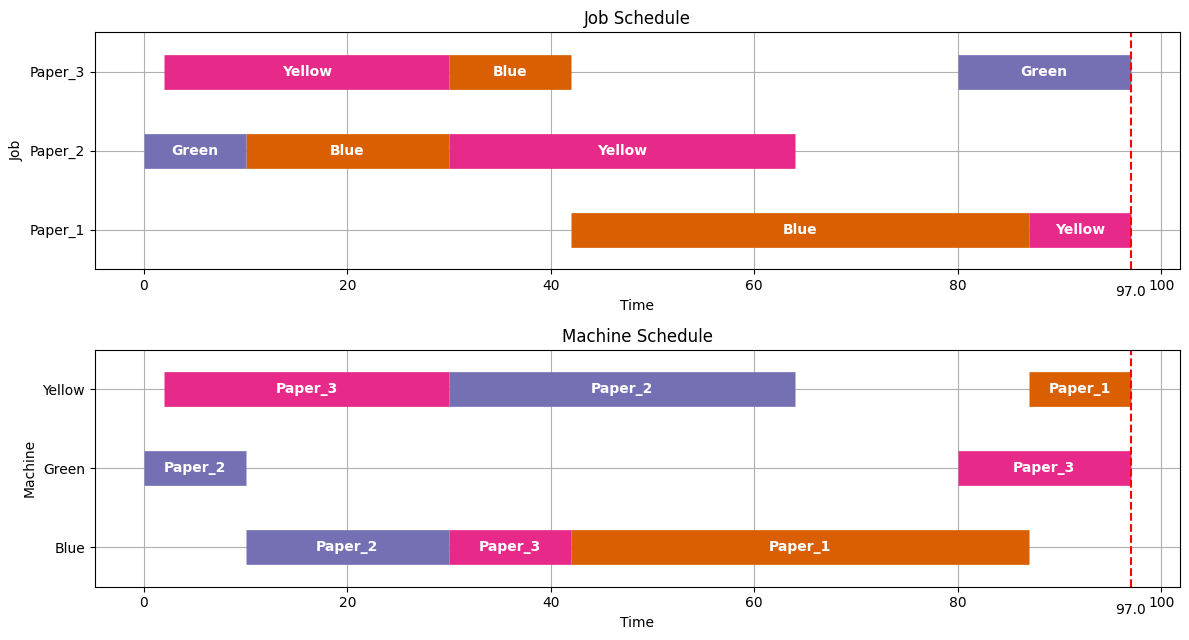## Application to the scheduling of batch processes#

We will now turn our attention to the application of the job shop scheduling problem to the short term scheduling of batch processes. We illustrate these techniques using Example II from Dunn (2013).Recipe

Mixer

Reactor

Separator

Packaging

A

1.0

5.0

4.0

1.5

B

-

-

4.5

1.0

C

-

3.0

5.0

1.5

### Single product strategies#

Before going further, we create a function to streamline the generation of the TASKS dictionary.

def recipe_to_tasks(jobs, machines, durations):
for j in jobs:
prec = (None, None)
for m, d in zip(machines, durations):
if prec == (None, None):
TASKS.update({(j, m): {"dur": d, "prec": None}})
else:
TASKS.update({(j, m): {"dur": d, "prec": prec}})

"A", ["Mixer", "Reactor", "Separator", "Packaging"], [1, 5, 4, 1.5]
)
visualize(jobshop(recipeA))

Solution determined by presolve;
objective minimize_makespan = 11.5.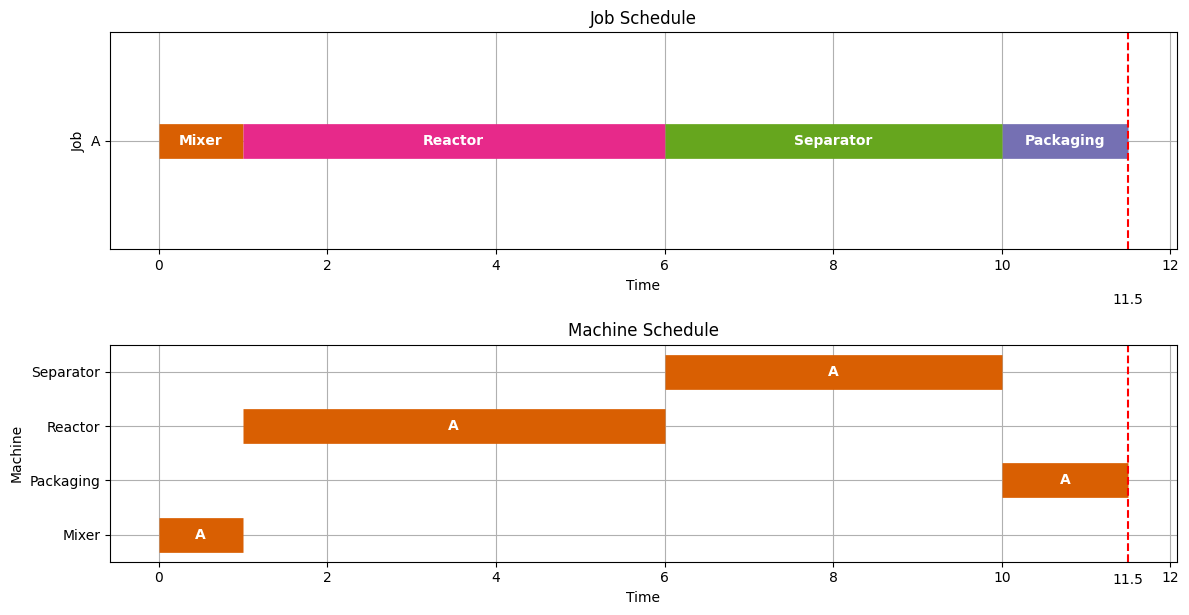recipeB = recipe_to_tasks("B", ["Separator", "Packaging"], [4.5, 1])
visualize(jobshop(recipeB))

Solution determined by presolve;
objective minimize_makespan = 5.5.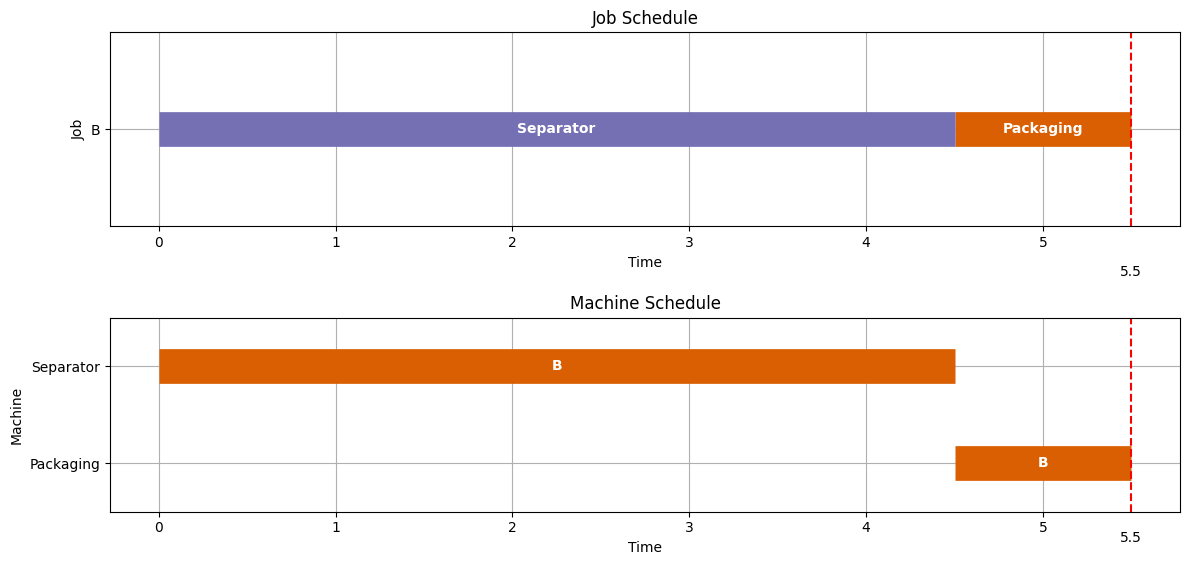recipeC = recipe_to_tasks("C", ["Separator", "Reactor", "Packaging"], [5, 3, 1.5])
visualize(jobshop(recipeC))

Solution determined by presolve;
objective minimize_makespan = 9.5.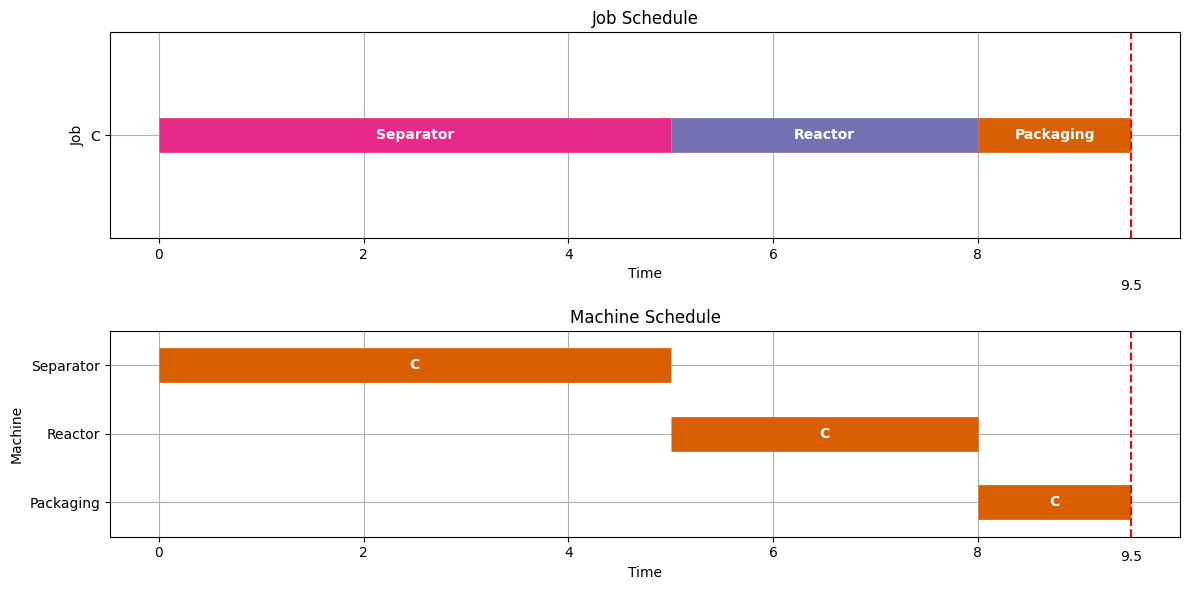Let’s now consider an optimal scheduling problem where we are wish to make two batches of Product A.

TASKS = recipe_to_tasks(
["A1", "A2", "A3", "A4"],
["Mixer", "Reactor", "Separator", "Packaging"],
[1, 5, 4, 1.5],
)
visualize(results)

HiGHS 1.5.1: HiGHS 1.5.1: optimal solution; objective 26.5
3411 simplex iterations
79 branching nodes
absmipgap=1e-06, relmipgap=3.77358e-08
Makespan = 26.5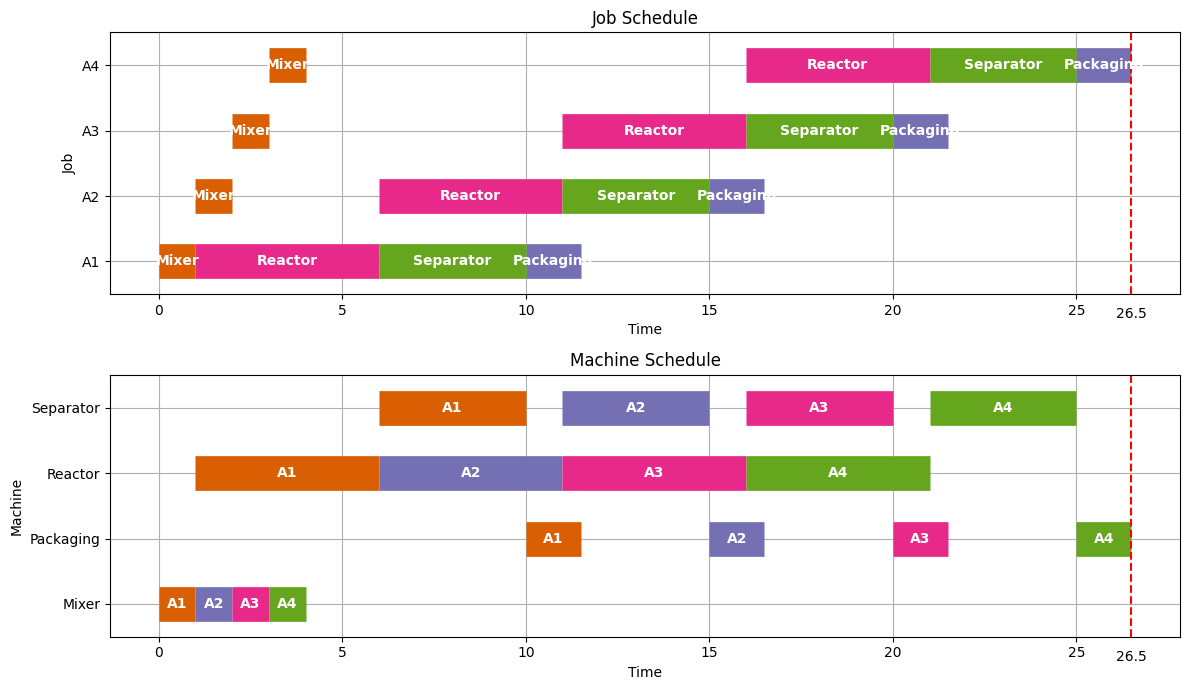Earlier we found that it took 11.5 hours to produce one batch of product A. As we see here, we can produce a second batch with only 5.0 additional hours because some of the tasks overlap. The overlapping of tasks is the key to gaining efficiency in batch processing facilities.

Let’s next consider production of a single batch each of products A, B, and C.

# update is used to append dictionaries

print(k, v)

visualize(results)

('A', 'Mixer') {'dur': 1, 'prec': None}
('A', 'Reactor') {'dur': 5, 'prec': ('A', 'Mixer')}
('A', 'Separator') {'dur': 4, 'prec': ('A', 'Reactor')}
('A', 'Packaging') {'dur': 1.5, 'prec': ('A', 'Separator')}
('B', 'Separator') {'dur': 4.5, 'prec': None}
('B', 'Packaging') {'dur': 1, 'prec': ('B', 'Separator')}
('C', 'Separator') {'dur': 5, 'prec': None}
('C', 'Reactor') {'dur': 3, 'prec': ('C', 'Separator')}
('C', 'Packaging') {'dur': 1.5, 'prec': ('C', 'Reactor')}
HiGHS 1.5.1: HiGHS 1.5.1: optimal solution; objective 15
71 simplex iterations
1 branching nodes
Makespan = 15.0The individual production of A, B, and C required 11.5, 5.5, and 9.5 hours, respectively, for a total of 25.5 hours. As we see here, by scheduling the production simultaneously, we can get all three batches done in just 15 hours.

As we see below, each additional set of three products takes an additional 13 hours. So there is considerable efficiency gained by scheduling over longer intervals whenever possible.

TASKS = recipe_to_tasks(
["A1", "A2"], ["Mixer", "Reactor", "Separator", "Packaging"], [1, 5, 4, 1.5]
)
recipe_to_tasks(["C1", "C2"], ["Separator", "Reactor", "Packaging"], [5, 3, 1.5])
)

visualize(results)

HiGHS 1.5.1:HiGHS 1.5.1: optimal solution; objective 28
12290 simplex iterations
622 branching nodes
Makespan = 27.99999999999888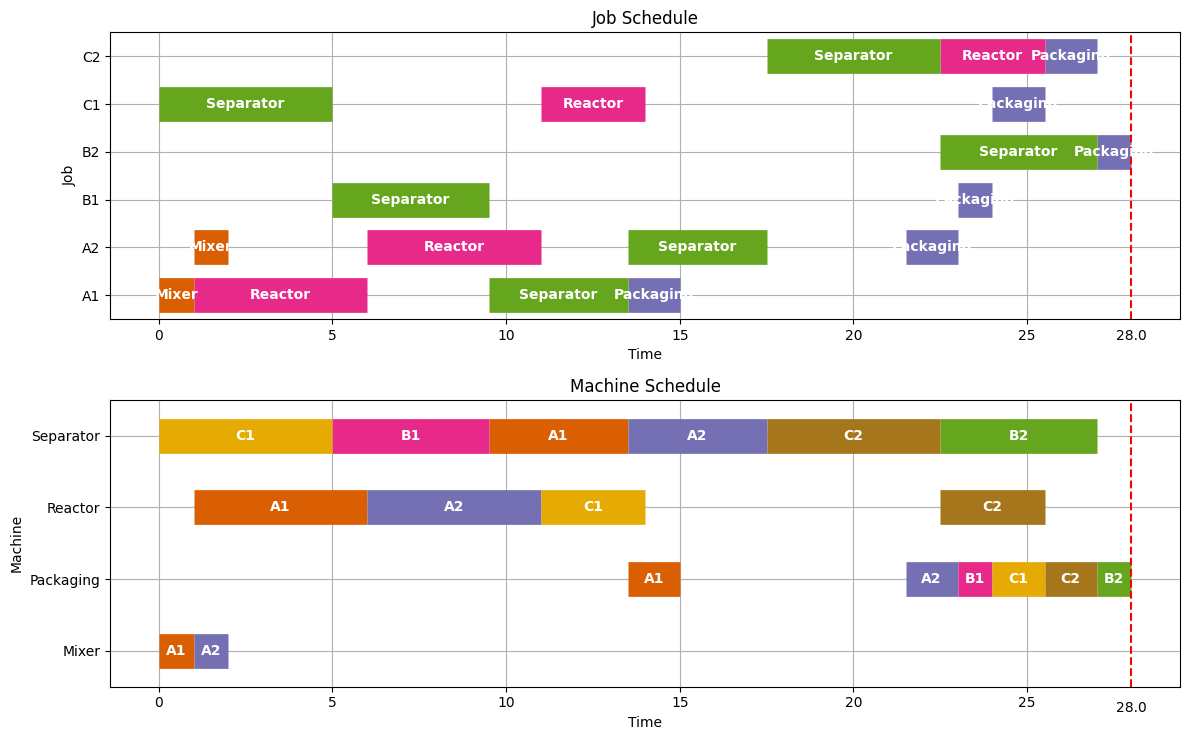### Adding time for unit clean out#

A common feature of batch unit operations is a requirement that equipment be cleaned prior to reuse.

In most cases the time needed for clean out would be specific to the equipment and product. But for the purposes this notebook, we implement can implement a simple clean out policy with a single non-negative parameter $$t_{clean} \geq 0$$ which, if specified, requires a period no less than $$t_{clean}$$ between the finish of one task and the start of another on every piece of equipment.

This policy is implemented by modifying the usual disjunctive constraints to avoid machine conflicts to read

\begin{align} \left[\text{start}_{j,m}+\text{dur}_{j,m} + t_{clean} \leq \text{start}_{k,m}\right] \vee \left[\text{start}_{k,m}+\text{dur}_{k,m} + t_{clean} \leq \text{start}_{j,m}\right] \end{align}

For this purpose, we write a new JobShopModel_Clean

%%writefile jobshop_model_clean.mod

set JOBS;
set MACHINES;
set DISJUNCTIONS within {JOBS, JOBS, MACHINES};

param ub;

var makespan >= 0, <= ub;
var start{TASKS} >= 0, <= ub;

minimize minimize_makespan: makespan;

start[j, m] + dur[j, m] <= makespan;

s.t. preceding {(j, m, k, n) in TASKORDER}:
start[k, n] + dur[k, n] <= start[j, m];

s.t. no_overlap {(j, k, m) in DISJUNCTIONS}:
(start[j, m] + dur[j, m] + tclean <= start[k, m]
or
start[k, m] + dur[k, m] + tclean <= start[j, m]);

Overwriting jobshop_model_clean.mod

def jobshop_model_clean(TASKS, tclean=0):
m = AMPL()

jobs = list(set([j for (j, m) in TASKS]))
machines = list(set([m for (j, m) in TASKS]))
(j, m, k, n)
if (k, n) == TASKS[(j, m)]["prec"]
]
disjunctions = [
(j, k, m) for (j, m) in tasks for (k, n) in tasks if m == n and j < k
]
ub = sum(dur[i] for i in dur)

m.set["JOBS"] = jobs
m.set["MACHINES"] = machines
m.set["DISJUNCTIONS"] = disjunctions

m.param["dur"] = dur
m.param["ub"] = ub

return m

results = jobshop_solve(model)
visualize(results)

HiGHS 1.5.1:HiGHS 1.5.1: optimal solution; objective 28
12290 simplex iterations
622 branching nodes
Makespan = 27.99999999999888### Adding a zero wait policy#

One of the issues in the use of job shop scheduling for chemical process operations are situations where there it is not possible to store intermediate materials. If there is no way to store intermediates, either in the processing equipment or in external vessels, then a zero-wait policy may be required.

A zero-wait policy requires subsequent processing machines to be available immediately upon completion of any task. To implement this policy, the usual precident sequencing constraint of a job shop scheduling problem, i.e.,

\begin{align*} \text{start}_{k,n}+\text{Dur}_{k,n} \leq \text{start}_{j,m}\ \ \ \ \text{for } (k,n) =\text{Prec}_{j,m} \end{align*}

is changed to

\begin{align*} \text{start}_{k,n}+\text{Dur}_{k,n} = \text{start}_{j,m}\ \ \ \ \text{for } (k,n) =\text{Prec}_{j,m}\text{ and ZW is True} \end{align*}

if the zero-wait policy is in effect.

While this could be implemented on an equipment or product specific basis, here we add an optional ZW flag to the JobShop function that, by default, is set to False.

%%writefile jobshop_model_clean_zw.mod

set JOBS;
set MACHINES;
set DISJUNCTIONS within {JOBS, JOBS, MACHINES};

param ub;
param tclean;
param ZW default 1;

var makespan >= 0, <= ub;
var start{TASKS} >= 0, <= ub;

minimize minimize_makespan: makespan;

start[j, m] + dur[j, m] <= makespan;

s.t. preceding {(j, m, k, n) in TASKORDER}:
ZW == 1 ==> start[k, n] + dur[k, n] == start[j, m]
else
start[k, n] + dur[k, n] <= start[j, m];

s.t. disjunctions {(j, k, m) in DISJUNCTIONS}:
(start[j, m] + dur[j, m] + tclean <= start[k, m]
or
start[k, m] + dur[k, m] + tclean <= start[j, m]);

Overwriting jobshop_model_clean_zw.mod

def jobshop_model_clean_zw(TASKS, tclean=0, ZW=False):
m = AMPL()

jobs = list(set([j for (j, m) in TASKS]))
machines = list(set([m for (j, m) in TASKS]))
(j, m, k, n)
if (k, n) == TASKS[(j, m)]["prec"]
]
disjunctions = [
(j, k, m) for (j, m) in tasks for (k, n) in tasks if m == n and j < k
]
ub = sum(dur[i] for i in dur)

m.set["JOBS"] = jobs
m.set["MACHINES"] = machines
m.set["DISJUNCTIONS"] = disjunctions

m.param["dur"] = dur
m.param["ub"] = ub
m.param["tclean"] = tclean
m.param["ZW"] = 1 if ZW else 0

m.option["solver"] = SOLVER

return m

results = jobshop_solve(model)
visualize(results)

HiGHS 1.5.1: HiGHS 1.5.1: optimal solution; objective 32
1552 simplex iterations
81 branching nodes
Makespan = 32.0